## Claus Martin Dachselt

ID:2041
First Name:Claus Martin
Last Name:Dachselt
Last Change:2021-04-10
Number of Files:34 (31st most prolific)

 Bernoulli's Differential Equation 1.4   (details) Prime ENG   2 KB / 3 KB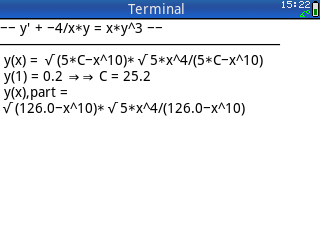Finds the solution to Bernoulli's Differential Equation given as: y' + g(x)*y =(x)*y^n, calculates the constant of integration C for any initial condition y(x0) = 0, if desired, and depicts the plot of the curve of y(x). By Claus Martin Dachselt. 2018-09-09

 Buckling Length of a Concrete Column 2.1   (details) 49/50 ENG   53 KB / 5 KB Determines for a concrete column with length Hi fixed to a system of beams and columns the effective (buckling) length on the basis of German Regulation DIN 1045-1 and "Heft" (Manual) 525 of the "Deutscher Ausschuss für Stahlbeton". Rel. 2.1 now includes an additional method of calculation and an easier way of input for the moments of inertia of the connected beams. Tested on 49g+ and 50g. By Claus Martin Dachselt. 2008-12-06

 Circle, Secant, Tangent   (details) Prime ENG   95 KB / 8 KB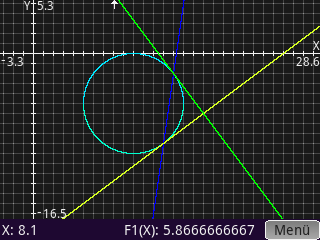Being given a circle C described by its center (xM,yM) and radius r in combination with a point P0(x0,y0) outside, Pc(xc,yc) inside or a secant defined by f(x), this CAS-program finds characteristic items like tangents T1,T2, tangential points P1, P2, chord between P1 and P2 and some more quantities. By Claus Martin Dachselt. 2019-02-11

 Conic Sections - Equation of 2nd Order 1.03   (details) Prime ENG   23 KB / 12 KB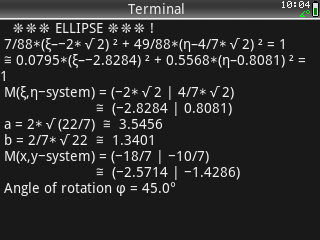Finds the type and characteristic items of conic sections, if the general equation of 2nd order is given in the form: a0*x² + b0*x*y + c0*y² + d0*x + e0*y + f0 = and draws the plot after calculation. By Claus Martin Dachselt. 2019-04-02

 Continuous Beam 2.01   (details) Prime ENG   189 KB / 19 KBCalculates the support moments of a continuous beam having different moments of inertia (Mb for a two-field span, Mb and Mc for a three-field beam). Several load cases for the same beam can be performed. Accepted are trapezoidal- and point loads, split in dead- and live load. By Claus Martin Dachselt. 2018-12-09

 Costs, Return, Profit 1.01   (details) Prime ENG   415 KB / 12 KBFrom defined preconditions the program finds the function of return R(x) (linear or quadratic function), the function of costs C(x) =*x^3 + b*x^2 +c*x + d and calculates characteristic properties of both of them and the profit function P(x) =(x)-C(x). By Claus Martin Dachselt. 2021-04-10

 Differential Equation of 4th and 3rd Order, Part 1 1.03   (details) Prime ENG   1,153 KB / 15 KB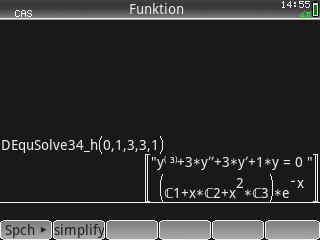Finds the solution y(x) = y_hom + y_part to the differential equation (D.E.) of 4th order a*y^(4) + b*y''' + c*y'' + d*y' + e*y = g(x) or of 3rd order b*y''' + c*y'' + d*y' + e*y = g(x), where a = 0. By Claus Martin Dachselt. 2020-12-30

 Differential Equation of 4th and 3rd Order, Part 2 1.02   (details) Prime ENG   1,125 KB / 10 KB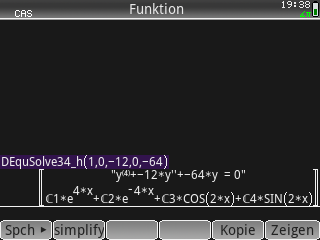Finds the solution y(x) = y_hom + y_part to the differential equation (D.E.) of 4th order a*y^(4) + b*y''' + c*y'' + d*y' + e*y = g(x) or of 3rd order (a = 0) b*y''' + c*y'' + d*y' + e*y = g(x), where g(x) = e^(alpha*x) * [c1*SIN(beta*x) + c2*COS(beta*x)]. By Claus Martin Dachselt. 2020-12-30

 Eclipses of Moon and Sun 1.0   (details) Prime ENG   5 KB / 14 KBFinds for a date given as yyyy.mmdd the next dates for full moon and new moon and checks for the occurence of a lunar and/or solar eclipse. If positive, some characteristics like kind of eclipse, magnitude and size of shadow in Earth radii ( E.R. ) are calculated. By Claus Martin Dachselt. 2018-02-27

 Ellipse, Secant, Tangents 1.02   (details) Prime ENG   99 KB / 9 KBBeing given an ellipse E described by its center (xM,yM), semi-major axis a and semi-minor axis b in combination with a point P0(x0,y0) outside, Pc(xc,yc) inside or a secant defined by f(x), this CAS-program finds characteristic items like tangents T1, T2, tangential points P1, P2, chord between P1 and P2 and some more quantities and draws the plot. By Claus Martin Dachselt. 2019-02-23

 Forced Oscillations 1.02   (details) Prime ENG   80 KB / 8 KB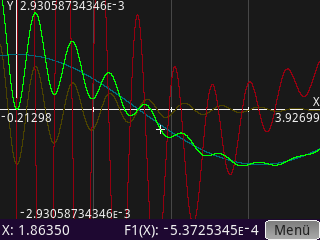Finds for the differential equation of a forced oscillation given as m*d2y/dt2 + d*dy/dt + c*y = 0*cos(omega*t) or m*d2y/dt2 + d*dy/dt + c*y = 0*sin(omega*t) or a combination of both the exact and approximate solution dependent on preset initial conditions t0, y0(t0), y'0(t0) and graphs the result. By Claus Martin Dachselt. 2019-12-29

 Framework - 2D and 3D 1.01   (details) Prime ENG   189 KB / 11 KBFinds by means of successive calculation the member forces S and their components Sx, Sy, Sz in a 2D- or 3D-truss. By Claus Martin Dachselt. 2018-12-09

 Function Defined by Preset Conditions 1.3   (details) Prime ENG   20 KB / 7 KB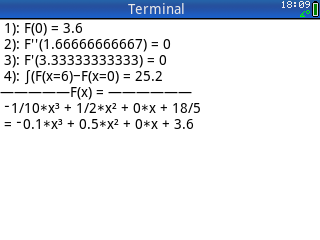Finds for a function f(x) of n-th degree ( n= 3 or 4 ) given as f(x) =*x^n + b*x^(n-1) + c*x^(n-2) + … the numerical values of the unknown coefficients a, b, c, … owing to preset conditions. These conditions may be: a given point P(x|y) as part of the graph of the function f(x), extremum or tangent in x for f´(x), point of inflexion in x as f´´(x)=- ( f´´(x)<>0 also possible ) - or the integral I (x) =nInt( f(x), x_lower, x_upper) between two points. After calculation, the graph F1(X) of the function is plotted. By Claus Martin Dachselt. 2018-08-18

 Geodetic Conversion between WGS84 and POTSDAM 1.03   (details) Prime ENG DEU   7 KB / 25 KBConverts between coordinates (UTM, sexagesimal or decimal degrees) of the WGS84 system to the corresponding coordinates (GAUSS-KRÜGER or degrees) in the POTSDAM system and vice versa, and finds the number of the German "Messtischblatt" including the marking of the 1/64 quadrant (8*8 subsquares). By Claus Martin Dachselt. 2021-04-10

 GK to Long/Lat Transformation 1.0   (details) 49/50 DEU   7 KB / 25 KB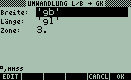The program converts for any point in Germany geographical coordinates given in longitude/latitude to Gauss-Krueger coordinates (German grid) and vice versa on the base of POTSDAM-date. Furthermore it shows the number of the topographical card ("Messtischblatt") and the corresponding quadrant (1 - 4). Tested on 49g+/50g (Vers. 2.09). By Claus Martin Dachselt. 2008-05-11

 Lagrange Interpolation 1.1   (details) Prime ENG   2 KB / 3 KB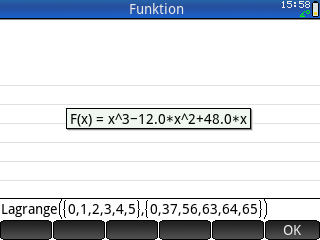Returns the interpolating Lagrange polynomial of minimum degree k for a set of points {x0, x1, …, xn}, {y0, y1, …, yn}, where 1 <= n-1 and n = 2, and depicts the plot of the function. By Claus Martin Dachselt. 2018-05-22

 Planes 1.2   (details) Prime ENG   57 KB / 14 KBFrom the field of Vector Algebra, finds for two planes, plane and straight line or plane and point typical properties like distance, point or line of intersection, angle between two planes. By Claus Martin Dachselt. 2018-05-22

 Point, Curve, Tangent 1.2   (details) Prime ENG   2 KB / 3 KBGiven a point (xP|yP) outside a curve described by f(x), this CAS program finds one or more tangent(s) Ti(x) through (xP|yP) to the curve, the accompanying tangential point(s) (xT|yT) on f(x) and the normal line(s) Ni(x) in (xT|yT). By Claus Martin Dachselt. 2018-04-09

 Polygonal Cross-Section 1.3   (details) Prime ENG   186 KB / 18 KB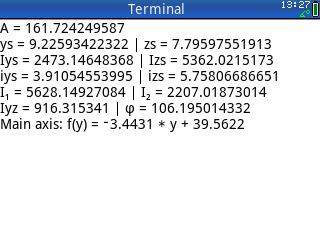Finds for any polygonal cross-section with gaps of circle areas the main section properties, draws the plot of the contour and computes for any load combination of N, My, Mz the stresses in all input points. Furthermore the intersection points of neutral axis ( Sigma = ) and contour of the polygon are calculated. By Claus Martin Dachselt. 2018-04-03

 REGBEM_1045 2.4   (details) 49/50 DEU   52 KB / 10 KB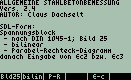This program determines for a rectangular concrete beam the amount of reinforcement As2 and/or As1 on the basis of German Regulation DIN 1045-1 given the dimensions, the class of concrete and the ratio As2/As1, which may be in the range between 0 and 1. Output are the strains on the section and the total amount of reinforcement on the basis of the chosen ratio. Optionally the net area of concrete (pressure zone less As2) may be considered. Tested on both the 49g+ and 50g. By Claus Martin Dachselt. 2009-06-27

 Reinforcement of a Concrete Beam 2.01   (details) Prime ENG DEU   108 KB / 19 KB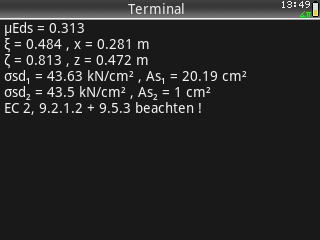Calculates on the basis of Eurocode 2 the tension reinforcement and, if required, the reinforcement in the compression zone of a concrete beam with given geometry, quality of concrete and loads M,Ed and N,Ed. Afterwards the calculation for a shear force V,Ed is performed. Documentation in German. By Claus Martin Dachselt. 2019-02-11

 Sectn Stress 1.0   (details) 49/50 ENG   73 KB / 3 KB After having calculated the characteristic properties of a cross section by means of program "Sectn48" (Library 1600) this program determines for a combination of longitudinal force and/or two moments Mx and My the stresses in every point of the structure and the location of the neutral axis. By Claus Martin Dachselt. 2008-05-11

 Single-Span Girder 1.2   (details) Prime ENG   248 KB / 19 KB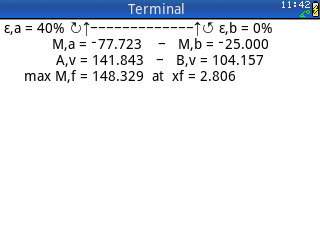Calculates for a single-span girder with any number of sections and elastic restraints or external moments at the supports the support reactions, end moments and maximum field moment including its location. Each section may have a trapezoidal load and a point load at the right end. Furthermore the shear force and the internal moment for any point of the beam can be calculated. By Claus Martin Dachselt. 2018-03-18

 Single-Span Girder 4.0   (details) 49/50 ENG   127 KB / 13 KBThe program determines for a single-span girder having restraints with reference to rotations at both ends the support moments, reaction forces and maximum field moment. The degrees of restraints may be in the range of 0 % to 100 %. Accepted are up to 25 trapezoidal knife-edged and individual loads. Now the combination of attached end-/cantilever moments and elastic restraint at the same support is provided. Tested for both the 49g+ and 50g. By Claus Martin Dachselt. 2009-06-27

 Soil Pressure of a Foundation with Open Gap 1.2   (details) Prime ENG   393 KB / 21 KBComputes for a rectangular foundation submitted to vertical loads N and moments My and Mz the soil pressure for all points of the contour in consideration of a open gap (no tension possible in the gap). The automatic consideration of the own weight is provided. A plot of the area of pressure is depicted after calculation. By Claus Martin Dachselt. 2018-04-29

 Spring Constant 1.2   (details) 49/50 ENG   11 KB / 2 KB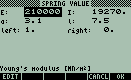This program determines the equivalent spring constants (vertical and rotational spring) of a one-field beam at any point of the length given its rigidity and the conditions of support at both ends. Tested on the HP 49g+ and 50g. By Claus Martin Dachselt. 2009-08-29

 Spring Constants 1.1   (details) Prime ENG   48 KB / 6 KB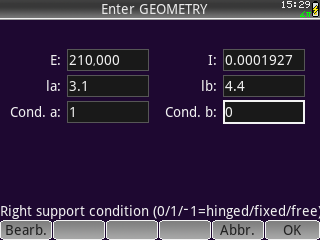Calculates for a one-field beam with modulus of elasticity E and moment of inertia I the equivalent spring constants (vertical spring c,v and rotational spring c,phi) at any point of the beam's length L. Different kinds of support conditions are provided. By Claus Martin Dachselt. 2020-04-11

 Straight Lines 1.2   (details) Prime ENG   52 KB / 10 KBFrom the field of Vector Algebra, finds for two straight lines L1 and L2 the distance between the lines, the points P1 and P2 of minimal distance on both lines, the intersection angle and checks, whether the lines are parallel, skew or have a common point of intersection. If the direction vector of L2 is entered as , the problem is handled as straight line L1 and point P. In this case the program finds the distance, the base point of perpendicular Pn, perpendicular vector P_ and the reflected point P0'. By Claus Martin Dachselt. 2018-05-22

 System of Two Linear Differential Equations 1.01   (details) Prime ENG   3 KB / 4 KB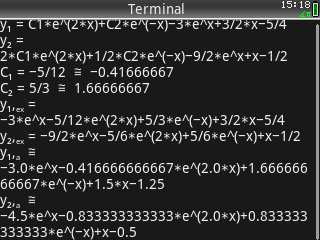Finds the solution to a system of two dífferential equations given as d(y1)/dx =*y1 + b*y2 + f(x) and d(y2)/dx =*y1 + d*y2 +g(x). For initial values x0, y1(x0), y2(x0) the constants of integration are calculated. A graph of both functions y1, y2 is provided. By Claus Martin Dachselt. 2018-12-09

 Tangent and Normal Line to a Curve Defined by f(x) 1.2   (details) Prime ENG   2 KB / 3 KB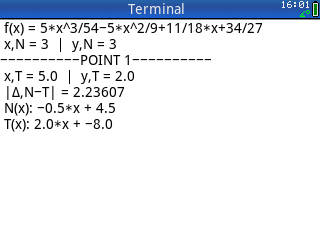This CAS program finds the normal (or perpendicular) line N(x) through a given point (xN,yN) to the curve of a given function f(x) and additionally computes the tangent in the common intersection point (xT,yT) of curve and N(x). By Claus Martin Dachselt. 2018-04-09

 Three-Edges-Supported Slab 3.1   (details) 49/50 ENG   53 KB / 19 KB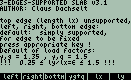This program determines for a two-way slab supported on the left, right, and bottom edge (top edge unsupported) the significant bending moments. The supports may be hinged and/or clamped in any order. Accepted load types are: area load q, triangle-shaped load qTri, and line load on top edge qR. The ratio ly/lx must be in the range of 0.25 and 1.5. Includes now variable load factors. Tested on both the 49g+ and 50g. By Claus Martin Dachselt. 2009-05-31

 Truss 2-D and 3-D 1.04   (details) Prime ENG   227 KB / 13 KBFinds the longitudinal forces in a 2-dimensional- or 3-dimensional truss by means of solving the matrix of equations of equilibrium at the nodes for the entire system. By Claus Martin Dachselt. 2019-02-23

 Two-Way Slab - Moments and Reactions 1.2   (details) 49/50 ENG   98 KB / 14 KBThe program determines for a two-way slab supported on all edges the moments and bearing reactions on the method of PIEPER/MARTENS. All kinds of support conditions (hinged and/or clamped in any order) are provided. It now offers a wider range for the ratio Ly/Lx and a more comfortable handling for compensation between neighboring restraint moments. For both the 49 and 50. By Claus Martin Dachselt. 2009-04-25

 UTM_GK Convert 2.3   (details) 49/50 DEU   54 KB / 14 KB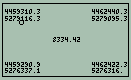Converts coordinates given in UTM (map datum: WGS 84) into those of the German Potsdam Datum (Gauss-Krueger or Longitude/Latitude) and vice versa. Additionally the numbers of the corresponding German "Messtischblatt" plus the accompanying quadrants/subquadrants are output. For the conversion using UTM values, the program Geodesia 1.03 (library number 1213) is required. For both the 49 and 50. By Claus Martin Dachselt. 2009-03-07

Part of the HP Calculator Archive,